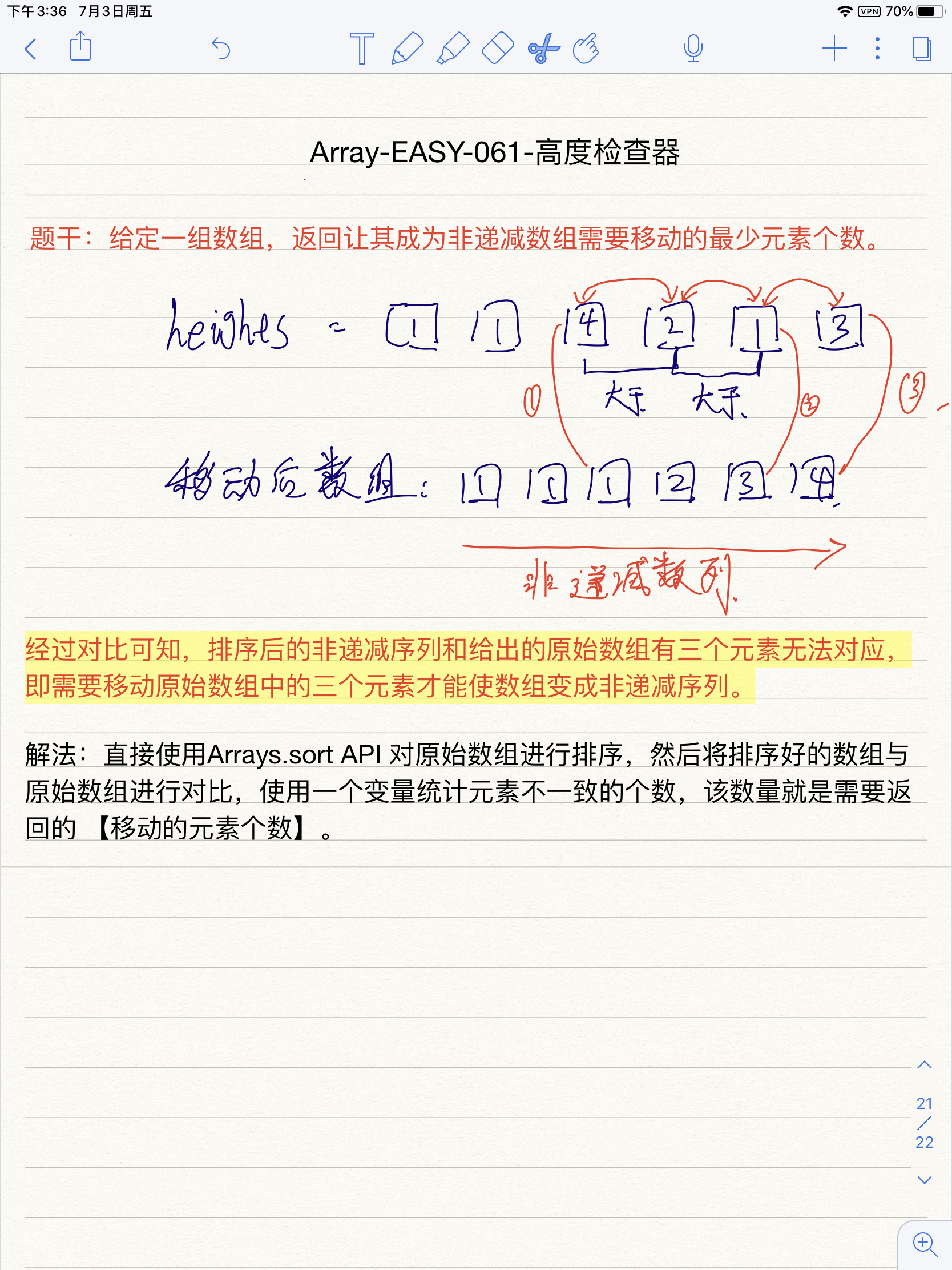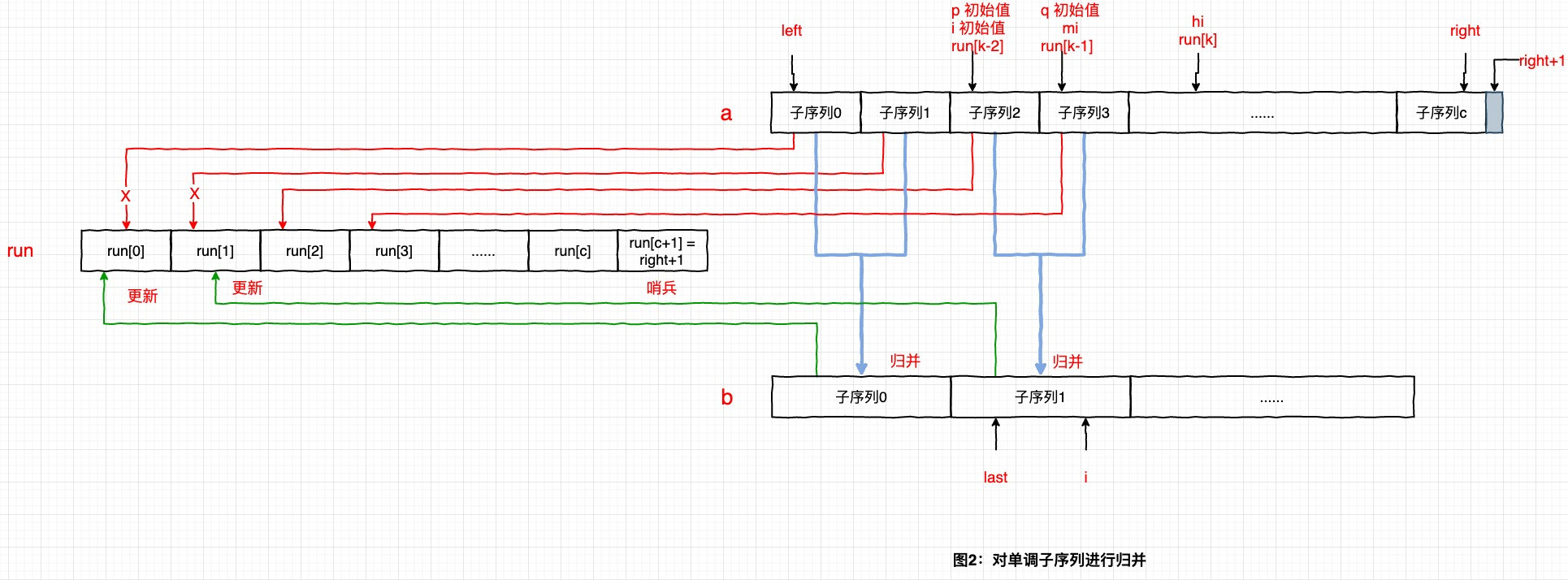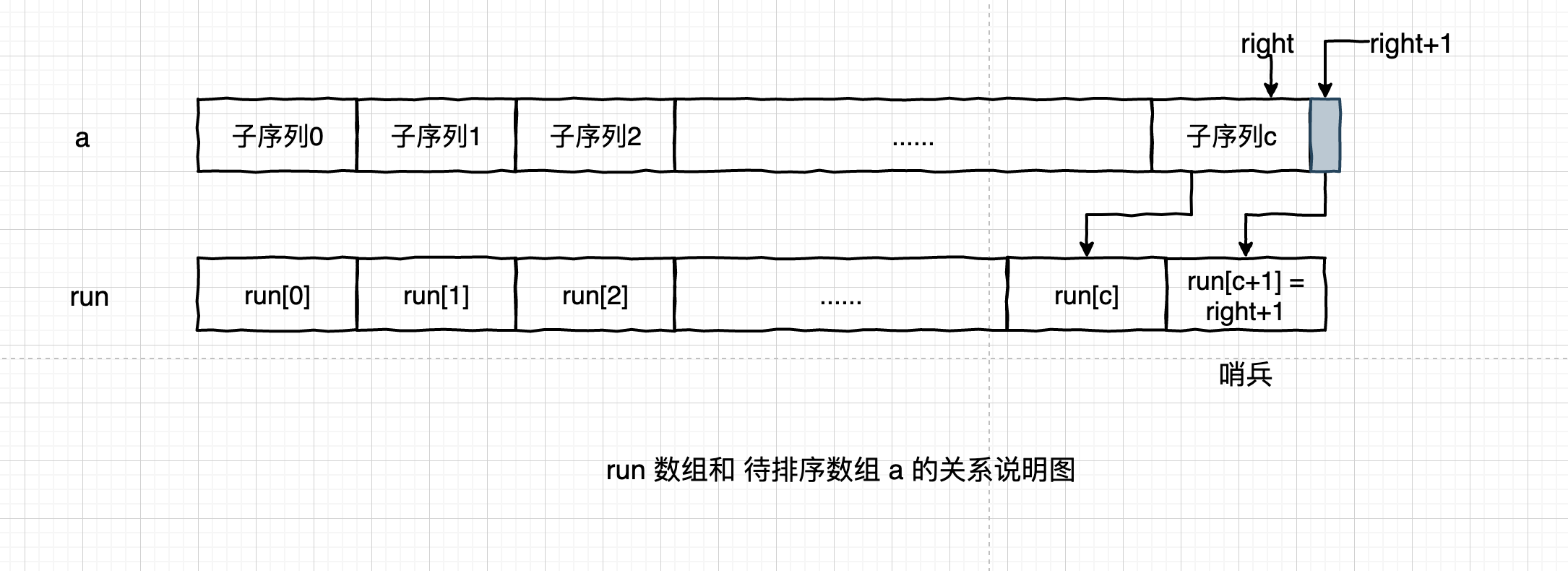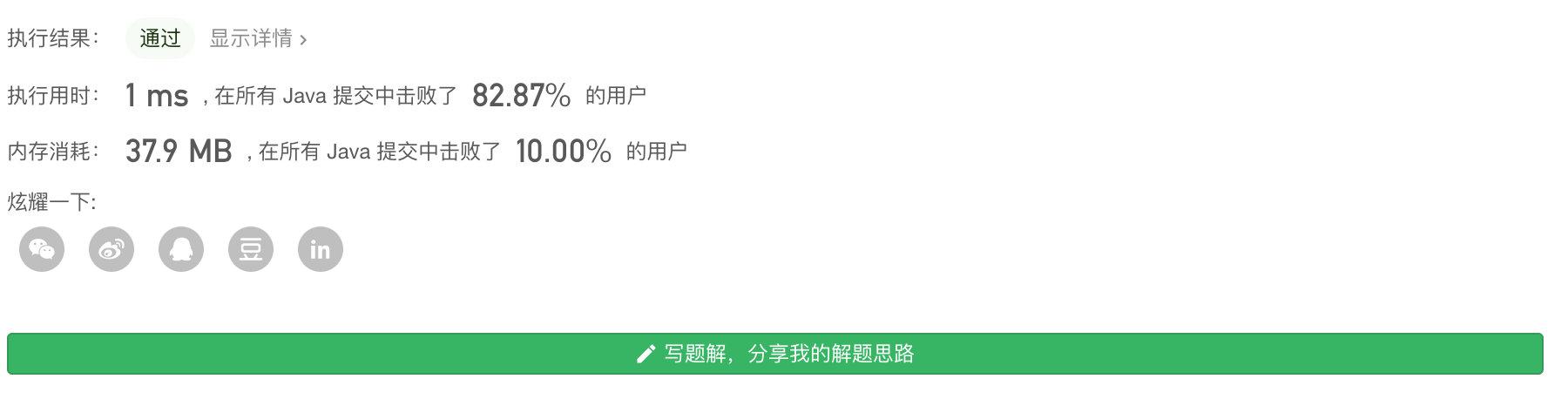### 示例：

输入：heights = [1,1,4,2,1,3]

当前数组：[1,1,4,2,1,3]
目标数组：[1,1,1,2,3,4]

1 <= heights.length <= 100
1 <= heights[i] <= 100

### 1. 读题

1、获得一个数组。

2、将这个数组变成非递减数组。

3、对比 原数组与非递减数组 之间有差异的元素的个数，这个数量就是需要移动的元素的数量。

### 2. 代码：

 public int heightChecker(int[] heights) {
int count = 0;
// 对原数组的副本进行排序
int[] temp = heights.clone();
// 对数组进行排序
Arrays.sort(temp);
// 遍历排序后的副本，计算原数组和排序后的数组中相同索引处不同的元素的个数
for (int i = 0; i < heights.length; i++) {
if (heights[i] != temp[i]) {
count++;
}
}
return count;
}


### 3.分析：

那么 Arrays.srot 是怎样实现的呢？

Arrays.sort 中的重载方法很多，但是我认为可以分为 3类

  // java.util.Arrays 中的 sort 方法

// ---基本类型数组排序方法 ---
void sort (char[])
void sort (char[], int, int)
void sort (byte[])
void sort (byte[], int, int)
void sort (double[])
void sort (float[], int, int)
void sort (float[])
void sort (int[], int, int)
void sort (long[])
void sort (long[], int, int)
void sort (long[])
void sort (short[], int, int)
void sort (short)
void sort (double[], int, int)

// --- 对象数组排序方法 ---
void sort (Object[])
void sort (Object[], int, int)

// --- 实现了自定义 Comparator 比较器的对象数组排序 ---
void sort (T[], Comparator)
void sort (T[], int, int, Comparator)



Arrays.sort 的排序效率O(log(n))

This algorithm offers O(n log(n)) performance on many data sets that cause other quicksorts to degrade to quadratic performance, and is typically faster than traditional (one-pivot) Quicksort implementations.


• Dual-Pivot Quicksort —— 双轴快速排序（不明觉厉）
• 如果数据集过多（many data sets) 将导致性能下降

sort 具体代码：

static void sort(int[] a, int left, int right,
int[] work, int workBase, int workLen) {
// a:传入的要进行排序的数组, left:0, right: a.length-1 ， work:nukk ,wrokebase 0, work len 0
// 其中 int[] work ,wokrBase,workLen 都是归并排序时需要用到的参数
// Use Quicksort on small arrays
// 可以看到，当 最后一个元素的索引小于 QUICKSORT_THRESHOLD 阈值 也就是 数组长度小于287的时候，直接使用快排对数组进行排序
// 第一部分，判断数组长度较小时，直接调用快速排序
if (right - left < QUICKSORT_THRESHOLD) {
// 调用快速排序算法
sort(a, left, right, true);
return;
}

/*
* Index run[i] is the start of i-th run
* (ascending or descending sequence).
*/
// 第二部分
//对数组的 「无序程度」 进行评估：对于任意数组，总是可以将其分割成若干个递增或递减的子数组，例如 {9,5,2,7,8,3,4} 可以拆分为 {9,5,2} {7,8},{3,4} 一个递减 两个递增数组
// 这里定义了 run 数组来存储每个子数组的开始的 「下标」，该部分的 for 循环体对于递增、递减、相等的 「子序列」 进行了处理 26、27 对递增子序列进行判断 ，28-32 对递减子序列进行判断
// MAX_RUN_COUNT -> 67， 也就是 run 数组的长度最大是 67
int[] run = new int[MAX_RUN_COUNT + 1];
// left == 0, right == a.lenght -1
int count = 0; run = left;

// Check if the array is nearly sorted
// //从left开始，遍历到right，这个count是把需要排序数组分成递增或者递减，相等的数组片段数量
for (int k = left; k < right; run[count] = k) {
// 对递增子序列进行处理
if (a[k] < a[k + 1]) { // ascending  如果前两个元素是递增的，就继续判断能递增到第几个元素
while (++k <= right && a[k - 1] <= a[k]);
} else if (a[k] > a[k + 1]) { // descending 如果前两个元素递减，就往后判断递减到第几个元素 ，有一点不同的是，这里把递减的片段改成了递增的了，方便后续进行归并排序（如果符合归并排序条件的话）
// 对递减子序列进行处理
while (++k <= right && a[k - 1] >= a[k]);
for (int lo = run[count] - 1, hi = k; ++lo < --hi; ) {
int t = a[lo]; a[lo] = a[hi]; a[hi] = t;
}
} else { // equal
// 如果相等序列的长度超过 MAX_RUN_LENGTH（33），则直接使用快排进行处理
for (int m = MAX_RUN_LENGTH; ++k <= right && a[k - 1] == a[k]; ) {
if (--m == 0) {
sort(a, left, right, true);
return;
}
}
}

/*
* The array is not highly structured,
* use Quicksort instead of merge sort.
*/
// 评估数组无序程度的指标：考察划分出子序列的个数，即 run 数组的大小 如果 run 数组的元素个数大于常量 MAX_RUN_COUNT（67），则认为整个数组是无序的，此时调用改进的快排算法进行排序，后面的代码不再执行
// 否则，认为程序基本是有序的，继续执行下面代码，利用「归并排序算法完成排序
if (++count == MAX_RUN_COUNT) {
sort(a, left, right, true);
return;
}
}

// Check special cases
// Implementation note: variable "right" is increased by 1.
// 当 run[count] 只 对应数组的最后一位时，扩展一位哨兵位
if (run[count] == right++) { // The last run contains one element
// 给 run 数组的末尾添加一个哨兵元素，这个元素的值 为 right+1 代表一个空子序列
run[++count] = right;
} else if (count == 1) { // The array is already sorted 当数组划分后只存在1个单调子序列时，说明数组本事就是有序的，不需要执行其他操作，程序结束。
return;
}

// Determine alternation base for merge
// 第三部分 ↓ 对划分好的数据进行双路归并排序，排序时用到了传入的 work[]
// 73 ~ 94 行是初始化的过程
byte odd = 0;
for (int n = 1; (n <<= 1) < count; odd ^= 1);

// Use or create temporary array b for merging
int[] b;                 // temp array; alternates with a
int ao, bo;              // array offsets from 'left'
int blen = right - left; // space needed for b
if (work == null || workLen < blen || workBase + blen > work.length) {
work = new int[blen];
workBase = 0;
}
if (odd == 0) {
System.arraycopy(a, left, work, workBase, blen);
b = a;
bo = 0;
a = work;
ao = workBase - left;
} else {
b = work;
ao = 0;
bo = workBase - left;
}

// Merging JDK 改进的 归并排序核心代码
// 99~ 121 行是归并排序的主体，循环的每一轮迭代都将 「数组a」 中的两个相邻的单调子序列「归并」为一个新的单调子序列，并将其存储在「数组b」中 ，102 行开始的内层 for循环 成对地归并「数组a」中的单调子序列，见图2
//last是归并完一次之后待归并的个数。count只要不是1，就证明还没有归并排序完成，就继续循环。
for (int last; count > 1; count = last) {
// 非要在这里才给 last 进行赋值，看着真别扭啊
// 两个一组进行归并排序
for (int k = (last = 0) + 2; k <= count; k += 2) {
int hi = run[k], mi = run[k - 1];
for (int i = run[k - 2], p = i, q = mi; i < hi; ++i) {
if (q >= hi || p < mi && a[p + ao] <= a[q + ao]) {
b[i + bo] = a[p++ + ao];
} else {
b[i + bo] = a[q++ + ao];
}
}
run[++last] = hi;
}
// 如果 a 数组中的子序列个数为奇数，则最后一个子序列无法进行配对、归并操作，此时直接将这个子序列复制到 b 中 对应代码 114 ~ 119 行
if ((count & 1) != 0) {
for (int i = right, lo = run[count - 1]; --i >= lo;
b[i + bo] = a[i + ao]
);
run[++last] = right;
}
// 每轮迭代后，子序列数目都会减少，反复地进行迭代归并后，最终整个数组只包含1个单调子序列，此时整个数组排序完成。
// 每轮迭代后，交换a、b指针，继续执行下一轮迭代，同样对a数组进行归并，存储在b数组中，利用a和b代表的存储空间反复地进行归并，最终就完成了数组的排序。
//交换指针对应下面2行代码
int[] t = a; a = b; b = t;
int o = ao; ao = bo; bo = o;
}
}### JDK 改进后的归并排序的思路：### 时间复杂度 & 空间复杂度：### 小结

JDK1.8 Arrays.sort 源码浅析

JDK源码解析(1)——数据数组排序：Arrays.sort()

Q.E.D.